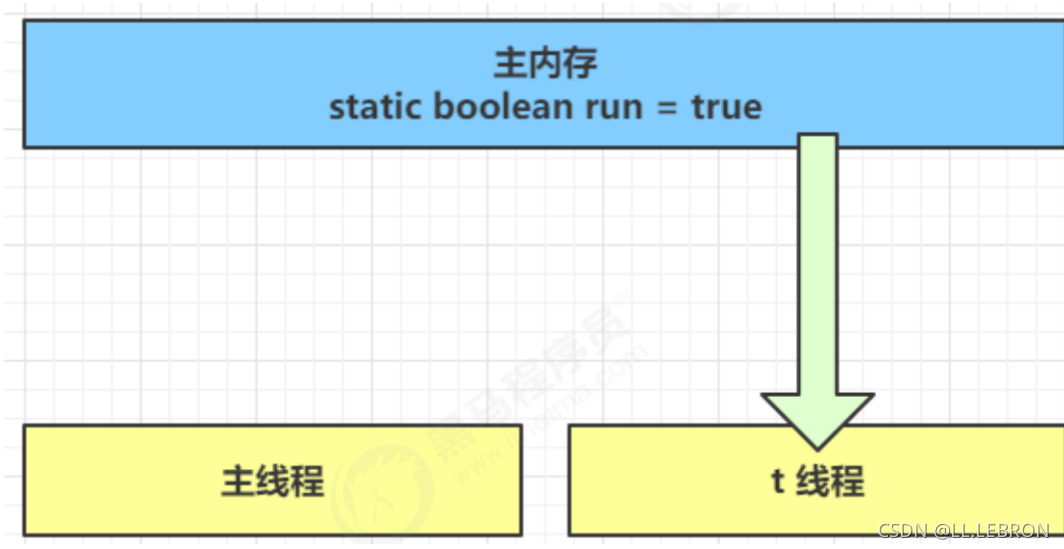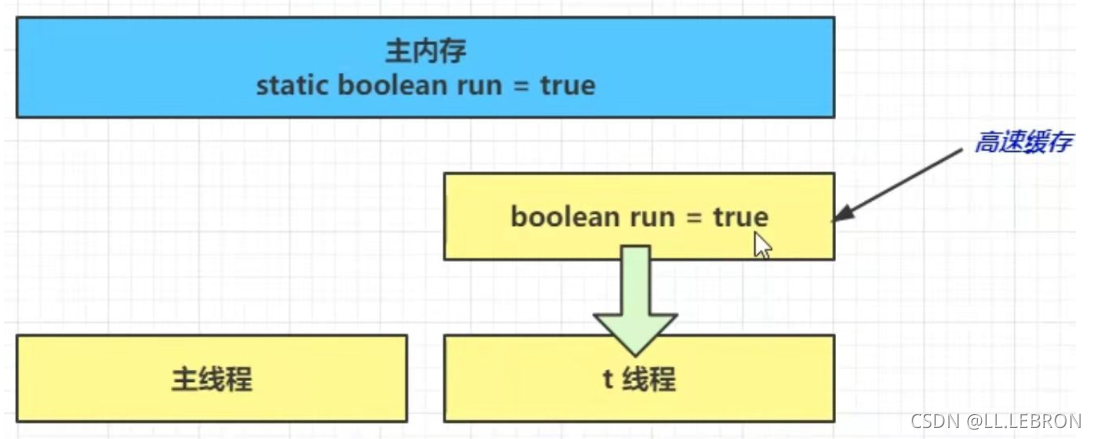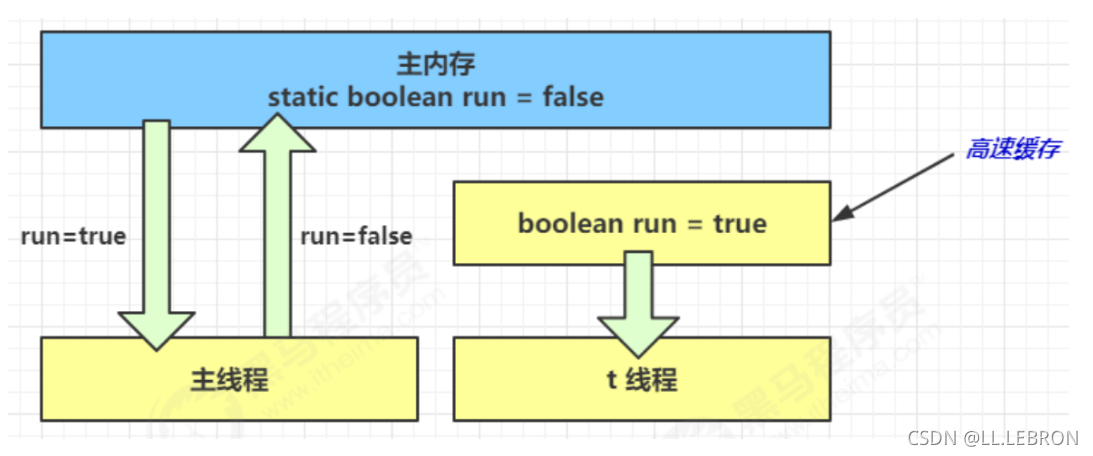# 【并发编程】

• 2022 年 5 月 14 日
• 本文字数：2387 字

阅读完需：约 8 分钟

while(run){

// ....

}

});

t.start();

run = false; // 线程 t 不会如预想的停下来

}

1. 初始状态， t 线程刚开始从主内存读取了 run 的值到工作内存1. 因为 t 线程要频繁从主内存中读取 run 的值，JIT 编译器会将 run 的值缓存至自己工作内存中的高速缓存中，减少对主存中 run 的访问，提高效率1. 1 秒之后，main 线程修改了 run 的值，并同步至主存，而 t 是从自己工作内存中的高速缓存中读取这个变量的值，结果永远是旧值• 使用 volatile（易变关键字）

• 它可以用来修饰成员变量静态成员变量，他可以避免线程从自己的工作缓存中查找变量的值，必须到主存中获取它的值，线程操作 volatile 变量都是直接操作主存

### [](()2-2 可见性 vs 原子性

• synchronized 语句块既可以保证代码块的原子性，也同时保证代码块内变量的可见性

• 但缺点是 synchronized 是属于重量级操作，性能相对更低。

• 如果在前面示例的死循环中加入 System.out.println() 会发现即使不加 volatile 修饰符，线程 t 也能正确看到对 run 变量的修改了，想一想为什么？

进入println源码，可以看出加了synchronized，保证了每次run变量都会从主存中获取


public void println(int x) {


    synchronized (this) {


        print(x);


        newLine();


    }


}


[](()3.有序性

### [](()3-1 诡异的结果

int num = 0;

boolean ready = false;

// 线程 1 执行此方法

public void actor1(I_Result r) {

r.r1 = num + num;

} else {

r.r1 = 1;

}

}

// 线程 2 执行此方法

public void actor2(I_Result r) {

num = 2;

}

### [](()3-2 解决方法

volatile 修饰的变量，可以禁用指令重排

@JCStressTest

@Outcome(id = {"1", "4"}, expect = Expect.ACCEPTABLE, desc = "ok")

@Outcome(id = "0", expect = Expect.ACCEPTABLE_INTERESTING, desc = "!!!!")

@State

public class ConcurrencyTest {

int num = 0;

volatile boolean ready = false;//可以禁用指令重排

@Actor

public void actor1(I_Result r) {

r.r1 = num + num;

} else {

r.r1 = 1;

}

}

@Actor

public void actor2(I_Result r) {

num = 2;

ready = true;《一线大厂 Java 面试题解析+后端开发学习笔记+最新架构讲解视频+实战项目源码讲义》无偿开源 威信搜索公众号【编程进阶路】}

}

### [](()3-3 有序性理解

static int i;

static int j;

// 在某个线程内执行如下赋值操作

i = ...; // 较为耗时的操作

j = ...;

i = ...; // 较为耗时的操作

j = ...;

j = ...;

i = ...; // 较为耗时的操作

### [](()3-4 happens-before

happens-before 规定了对共享变量写操作对其它线程的读操作可见，它是可见性有序性的一套规则总结，抛开以下 happens-before 规则，JMM 并不能保证一个线程对共享变量的写，对于其它线程对该共享变量的读可见

• 线程解锁 m 之前对变量的写，对于接下来对 m 加锁的其它线程对该变量的读可见

static int x;

static Object m = new Object();

synchronized(m) {

x = 10;

}

},"t1").start();

synchronized(m) {

System.out.println(x);

}

},"t2").start()

• 线程对 volatile 变量的写，对接下来其它线程对该变量的读可见

volatile static int x;

x = 10;

},"t1").start();

System.out.println(x);

},"t2").start();

• 线程 start 前对变量的写，对该线程开始后对该变量的读可见

static int x;

x = 10;

System.out.println(x);

},"t2").start();

• 线程结束前对变量的写，对其它线程得知它结束后的读可见（比如其它线程调用 t1.isAlive()t1.join()等待它结束）

static int x;

x = 10;

},"t1");

t1.start();

t1.join();

System.out.println(x);

• 线程 t1 打断 t2（interrupt）前对变量的写，对于其他线程得知 t2 被打断后对变量的读可见（通 过t2.interruptedt2.isInterrupted

static int x;

public static void main(String[] args) {

while(true) {

System.out.println(x);//10

break;

}

}

},"t2");

t2.start();

try {

} catch (InterruptedException e) {

e.printStackTrace();

}

x = 10;

t2.interrupt();

},"t1").start();

while(!t2.isInterrupted()) {

}

System.out.println(x);//10

}

• 对变量默认值（0，false，null）的写，对其它线程对该变量的读可见

• 具有传递性，如果 x hb-> y 并且 y hb-> z 那么有 x hb-> z

volatile static int x;

static int y;

y = 10;

x = 20;//写屏障，y 也会同步到主存

},"t1").start();

// x=20 对 t2 可见, 同时 y=10 也对 t2 可见

System.out.println(x);

},"t2").start();## 评论